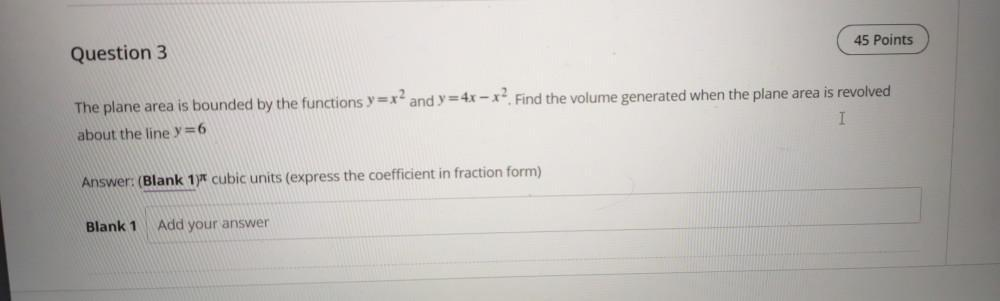Question:

# The plane area is bounded by the functions y=x^2 and y=4x - x^2 Find the volume generated when the plane area is revolved about the line y=6The plane area is bounded by the functions y=x^2 and y=4x - x^2 Find the volume generated when the plane area is revolved about the line y=6